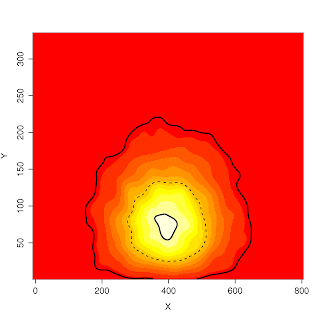## Tuesday, March 18, 2008

### Plotting contoursPlenty of packages allow you to plot contours of a "z" value; however, I wanted to be able to plot a specific density contour of a sample from a bivariate distribution over a plot that was a function of the x and y parameters. The example only plots the density of x and y; however, if you set plot.dens=FALSE, the contours will be added to the active display device (i.e., over an image(x,y,function(x,y){...})).

This function also lets you specify the line widths and types for each of the contours.
`############################################# drawcontour.R## Written by J.D. Forester, 17 March 2008#############################################This function draws an approximate density contour based on##empirical, bivariate data.##change testit to FALSE if sourcing the filetestit=TRUEdraw.contour<-function(a,alpha=0.95,plot.dens=FALSE, line.width=2, line.type=1, limits=NULL, density.res=300,spline.smooth=-1,...){  ##a is a list or matrix of x and y coordinates (e.g., a=list("x"=rnorm(100),"y"=rnorm(100)))  ## if a is a list or dataframe, the components must be labeled "x" and "y"  ## if a is a matrix, the first column is assumed to be x, the second y  ##alpha is the contour level desired  ##if plot.dens==TRUE, then the joint density of x and y are plotted,  ##   otherwise the contour is added to the current plot.  ##density.res controls the resolution of the density plot  ##A key assumption of this function is that very little probability mass lies outside the limits of  ## the x and y values in "a". This is likely reasonable if the number of observations in a is large.    require(MASS)  require(ks)  if(length(line.width)!=length(alpha)){    line.width <- rep(line.width,length(alpha))  }  if(length(line.type)!=length(alpha)){    line.type <- rep(line.type,length(alpha))  }    if(is.matrix(a)){    a=list("x"=a[,1],"y"=a[,2])  }  ##generate approximate density values  if(is.null(limits)){    limits=c(range(a\$x),range(a\$y))  }  f1<-kde2d(a\$x,a\$y,n=density.res,lims=limits)  ##plot empirical density  if(plot.dens) image(f1,...)    if(is.null(dev.list())){    ##ensure that there is a window in which to draw the contour    plot(a,type="n",xlim=limits[1:2],ylim=limits[3:4],...)  }      ##estimate critical contour value  ## assume that density outside of plot is very small  zdens <- rev(sort(f1\$z))  Czdens <- cumsum(zdens)  Czdens <- (Czdens/Czdens[length(zdens)])  for(cont.level in 1:length(alpha)){    ##This loop allows for multiple contour levels    crit.val <- zdens[max(which(Czdens<=alpha[cont.level]))]       ##determine coordinates of critical contour    b.full=contourLines(f1,levels=crit.val)    for(c in 1:length(b.full)){      ##This loop is used in case the density is multimodal or if the desired contour      ##  extends outside the plotting region      b=list("x"=as.vector(unlist(b.full[[c]])),"y"=as.vector(unlist(b.full[[c]])))            ##plot desired contour      line.dat<-xspline(b,shape=spline.smooth,open=TRUE,draw=FALSE)      lines(line.dat,lty=line.type[cont.level],lwd=line.width[cont.level])    }  }}################################Test the function################################generate dataif(testit){  n=10000  a<-list("x"=rnorm(n,400,100),"y"=rweibull(n,2,100))  draw.contour(a=a,alpha=c(0.95,0.5,0.05),line.width=c(2,1,2),line.type=c(1,2,1),plot.dens=TRUE, xlab="X", ylab="Y")}`